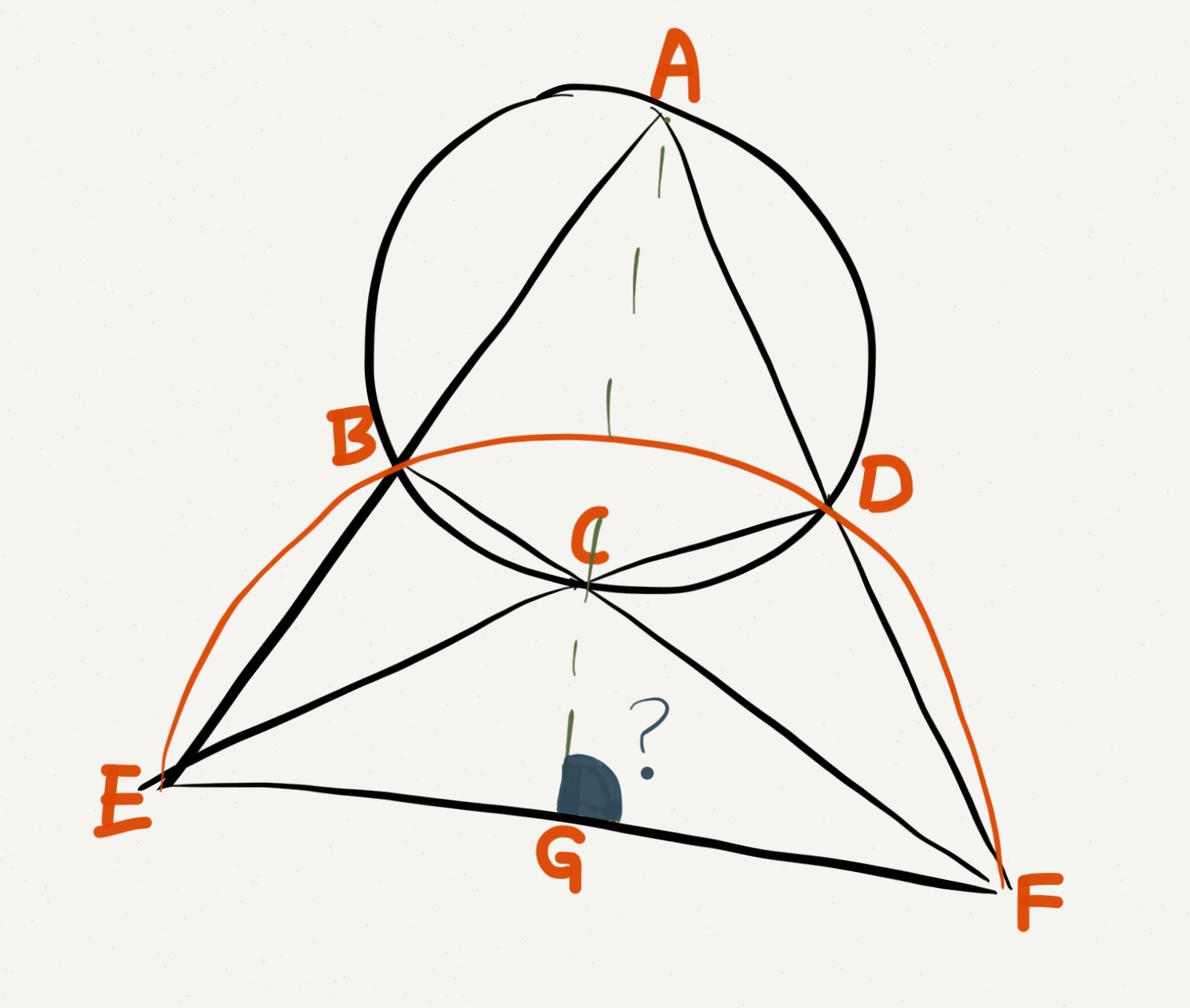# Day 21: This Diagram Looks FamiliarA cyclic quadrilateral $ABCD$ has its sides extended and they do meet, creating points $E$ and $F$ in the diagram.

As shown, $B, D, E, F$ are also concyclic.

Find the angle between line segments $EF$ and $AC$.

×